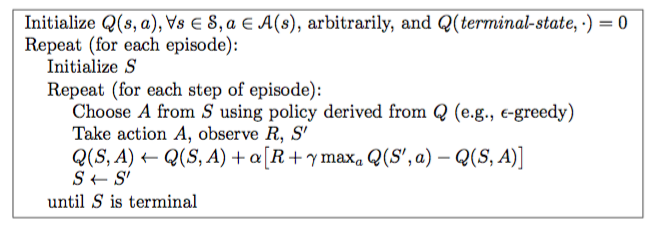# Learning motor primitives on a small crawling robot using Q-Learning.¶

Github link to the full project: Autonomous_robot_project

NB viewer link for this notebook: Learning motor primitives on a small crawling robot using Q-Learning

Binder version: Learning motor primitives on a small crawling robot using Q-Learning: go to Code\Simulation\Crawling_Simulation.ipynb

## Table of content:¶

In :
# -*- coding: utf-8 -*-
import re
import time
import datetime
import operator
import numpy as np
import pandas as pd
import collections
import unicodedata
import collections
import seaborn as sns
import collections
import matplotlib.pylab as pylab
import matplotlib.pyplot as plt

from tqdm import tqdm
from collections import Counter
from datetime import datetime, date, timedelta
from IPython.display import Image

%matplotlib inline

pylab.rcParams['figure.figsize'] = 11,8

plt.style.use('bmh')
colors = ['#348ABD', '#A60628', '#7A68A6', '#467821', '#D55E00',
'#CC79A7', '#56B4E9', '#009E73', '#F0E442', '#0072B2']


## Review of Q-learning ¶

### 1. Temporal-Difference learning:¶

Temporal-difference learning can be considered as one of the most important techniques in reinforcement learning. It combines 2 big ideas of Monte Carlo method and Dynamic Programming: the agent learns directly from raw experience without a model of the environment and it updates its estimates immediately without waiting for the final outcome. This method is ideal for the on-line, incremental situation (crawling robot, autonomous vehicles,...).

The basic TD method, known as TD(0), can be expressed with the formula:

$v(S_t) \leftarrow v(S_t) + \alpha \left [ R_{t+1} + \gamma v(S_{t+1}) - v(S_t) \right ]$

Here we can see that the algorithm updates the estimate value function $v(S_t)$ immediately in the next time step using $v(S_{t+1})$, which is very different to the classic Monte Carlo method which need to wait to the end of the simulation and update using the final return $G_t$.

$v(S_t) \leftarrow v(S_t) + \alpha \left [ G_t - v(S_t) \right ]$

### 2. Q-learning Algorithm:¶

The above TD prediction technique is used in Q-learning to solve the control problem (finding the best policy that optimises the future outcome. ). The main algorithm is define as:

$Q(S_t,A_t) \leftarrow Q(S_t, A_t) + \alpha \left [ R_{t+1} + \gamma \max_a Q(S_{t+1},a) - Q(S_t,A_t) \right ]$

The algorithm can be then implemented as follows:

In :
Image(filename="img/q-learning.png")

Out:(Source: Reinforcement Learning - An Introduction.)

### 3. Behavior policy¶

Q-learning is an off-policy learning method. The algorithm has 2 policies: one used to choose the next action in each time step, called behavior policy, and one whose value function we seek to learn, called the target policy. The choice of behavior policy is important as it is the main way to cope with the exploitation-exploration dilemma.

In this project, we have considered 2 types of behavior policy: $\epsilon$-greedy and the Boltzmann selection.

#### 3.1 $\epsilon$-greedy selection¶

When employing this strategy, the agent will usually follow the greedy rule, which means that it will chose the best action according to the state-action values. However, there is a small probability $\epsilon$ that, rather than take the best action, the agent will uniformly select an action from the remaining actions.

#### 3.2 Boltzmann selection¶

With Boltzmann selection, the relative value of the state-action values is taken into account. By using a Softmax function, we "squash" the vector of state-action values into a vector of "probability", i.e it sums to 1. The action will be sampled from this vector with the corresponding probability.

Concretely, at a state s, an action a is selected with probability:

$p = \frac{\exp(\frac{Q(s,a) - max_bQ(s,b)}{T})}{ \sum_{a} \exp(\frac{Q(s,a) - max_bQ(s,b)}{T})}$

T is called the temperature, and increases the exploration rate as it increases.

Later, in the parameter optimisation section, we will examine the difference in performance between these 2 policies.

## Robot simulation ¶

To analyse the performance of the algorithm, we have created a crawling robot simulation of our robotics project. The goal of this project is to train the robot to move forward using reinforcement learning.

The real life robot has 2 servomotors for locomotion and 2 freewheels with encoders to reduce friction and measure displacement. The chassis and wheels with encoders can simply be taken from a previous project (Raspberry Pi line tracker). As for the motors, we have a large supply of dynamixel XL-320 which are powerful enough to lift the robot’s arm and drag it along the ground.The arm’s parts were 3D printed on site at Eirlab, the school’s fab lab.

Another important reason we are starting with this simple design is that it allows us to quickly and easily test the basic theory for Q learning. Since the robot only has 2 degrees of freedom, the complexity of the algorithm will be drastically reduced compared to our final design. We have allowed each servo to have a $120^{\circ}$ range of motion split up in 7 discrete positions $20^{\circ}$ apart.

In :
Image(filename="img/robot1bras-2.jpg", width=600, height=600)

Out: